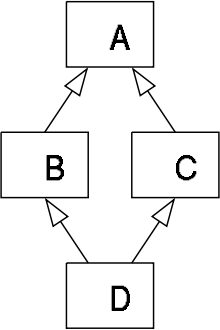# Method resolution order in Python Inheritance

• Difficulty Level : Basic
• Last Updated : 25 Mar, 2022

Method Resolution Order :
Method Resolution Order(MRO) it denotes the way a programming language resolves a method or attribute. Python supports classes inheriting from other classes. The class being inherited is called the Parent or Superclass, while the class that inherits is called the Child or Subclass. In python, method resolution order defines the order in which the base classes are searched when executing a method. First, the method or attribute is searched within a class and then it follows the order we specified while inheriting. This order is also called Linearization of a class and set of rules are called MRO(Method Resolution Order). While inheriting from another class, the interpreter needs a way to resolve the methods that are being called via an instance. Thus we need the method resolution order. For Example

## Python3

 `# Python program showing``# how MRO works` `class` `A:``    ``def` `rk(``self``):``        ``print``(" In ``class` `A")``class` `B(A):``    ``def` `rk(``self``):``        ``print``(" In ``class` `B")` `r ``=` `B()``r.rk()`

Output:

` In class B`

In the above example the methods that are invoked is from class B but not from class A, and this is due to Method Resolution Order(MRO).
The order that follows in the above code is- class B – > class A
In multiple inheritances, the methods are executed based on the order specified while inheriting the classes. For the languages that support single inheritance, method resolution order is not interesting, but the languages that support multiple inheritance method resolution order plays a very crucial role. Let’s look over another example to deeply understand the method resolution order:

## Python3

 `# Python program showing``# how MRO works` `class` `A:``    ``def` `rk(``self``):``        ``print``(" In ``class` `A")``class` `B(A):``    ``def` `rk(``self``):``        ``print``(" In ``class` `B")``class` `C(A):``    ``def` `rk(``self``):``        ``print``("In ``class` `C")` `# classes ordering``class` `D(B, C):``    ``pass``   ` `r ``=` `D()``r.rk()`

Output:

` In class B`

In the above example we use multiple inheritances and it is also called Diamond inheritance and it looks as follows:Python follows a depth-first lookup order and hence ends up calling the method from class A. By following the method resolution order, the lookup order as follows.
Class D -> Class B -> Class C -> Class A
Python follows depth-first order to resolve the methods and attributes. So in the above example, it executes the method in class B.

Old and New Style Order :
In the older version of Python(2.1) we are bound to use old-style classes but in Python(3.x & 2.2) we are bound to use only new classes. New style classes are the ones whose first parent inherits from Python root ‘object’ class.

## Python3

 `# Old style class``class` `OldStyleClass:``    ``pass` `# New style class``class` `NewStyleClass(``object``):``    ``pass`

Method resolution order(MRO) in both the declaration style is different. Old style classes use DLR or depth-first left to right algorithm whereas new style classes use C3 Linearization algorithm for method resolution while doing multiple inheritances.

DLR Algorithm
During implementing multiple inheritances, Python builds a list of classes to search as it needs to resolve which method has to be called when one is invoked by an instance. As the name suggests, the method resolution order will search the depth-first, then go left to right. For Example

## Python3

 `class` `A:``    ``pass`  `class` `B:``    ``pass`  `class` `C(A, B):``    ``pass`  `class` `D(B, A):``    ``pass`  `class` `E(C,D):``    ``pass`

In the above Example algorithm first looks into the instance class for the invoked method. If not present, then it looks into the first parent, if that too is not present then-parent of the parent is looked into. This continues till the end of the depth of class and finally, till the end of inherited classes. So, the resolution order in our last example will be D, B, A, C, A. But, A cannot be twice present thus, the order will be D, B, A, C. But this algorithm varying in different ways and showing different behaviours at different times .So Samuele Pedroni first discovered an inconsistency and introduce C3 Linearization algorithm.

C3 Linearization Algorithm :
C3 Linearization algorithm is an algorithm that uses new-style classes. It is used to remove an inconsistency created by DLR Algorithm. It has certain limitation they are:

• Children precede their parents
• If a class inherits from multiple classes, they are kept in the order specified in the tuple of the base class.

C3 Linearization Algorithm works on three rules:

• Inheritance graph determines the structure of method resolution order.
• User have to visit the super class only after the method of the local classes are visited.
• Monotonicity

Methods for Method Resolution Order(MRO) of a class:
To get the method resolution order of a class we can use either __mro__ attribute or mro() method. By using these methods we can display the order in which methods are resolved. For Example

## Python3

 `# Python program to show the order``# in which methods are resolved` `class` `A:``    ``def` `rk(``self``):``        ``print``(" In ``class` `A")``class` `B:``    ``def` `rk(``self``):``        ``print``(" In ``class` `B")` `# classes ordering``class` `C(A, B):``    ``def` `__init__(``self``):``        ``print``("Constructor C")` `r ``=` `C()` `# it prints the lookup order``print``(C.__mro__)``print``(C.mro())`

Output:

```Constructor C

(<class '__main__.C'>, <class '__main__.A'>, <class '__main__.B'>, <class 'object'>)

[<class '__main__.C'>, <class '__main__.A'>, <class '__main__.B'>, <class 'object'>]```

My Personal Notes arrow_drop_up